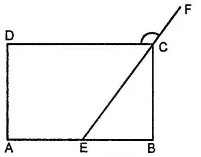Find the measure of ∠P and ∠S, if bar $$\overline {SP}|| \overline {RQ}$$ in the following figure. (If you find m∠R, is there more than one method to find m∠P )Asked by Aaryan | 1 year ago |  140

##### Solution :-

∠ P + ∠ Q = 180° (Angles on the same side of transversal)

∠ P + 130° = 180°

∠ P = 50°

∠ R + ∠ S = 180° (Angles on the same side of transversal)

90° + ∠ R = 180°

∠ S = 90°

Yes. There is one more method to find the measure of m∠ P.

m∠ R and m∠ Q are given. After finding m∠ S, the angle sum property of a quadrilateral can be applied to find m∠ P.

Answered by Sakshi | 1 year ago

### Related Questions

#### In the given figure, PQRS is a kite. Find the values of x and y.

In the given figure, PQRS is a kite. Find the values of x and y.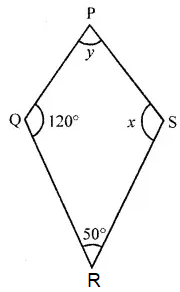#### In the given isosceles trapezium ABCD, ∠C = 102°. Find all the remaining angles of the trapezium.

In the given isosceles trapezium ABCD, ∠C = 102°. Find all the remaining angles of the trapezium.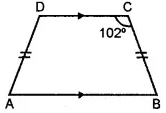#### In the given figure, ABCD is a rhombus and ∠ABD = 50°. Find :

In the given figure, ABCD is a rhombus and ∠ABD = 50°. Find :

(i) ∠CAB

(ii) ∠BCD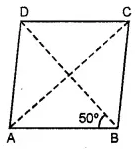#### In the given figure, ABCD is a rectangle and diagonals intersect at O. If ∠AOB = 118°, find

In the given figure, ABCD is a rectangle and diagonals intersect at O. If ∠AOB = 118°, find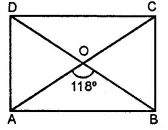(i) ∠ABO

(iii) ∠OCB